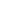# Ancient Mathematics from Egypt to Babylon

\$75 Limited inc GST / \$68
Ancient Mathematics from Egypt to Babylon

<p>By studying ancient mathematical artefacts, such as Rhind papyrus, we will see the ancient system of counting, arithmetic operations and geometry. Egyptians started with measuring parts of land

...
Enrol into Ancient Egypt and Babylonian mathematics; Ancient Greek mathematics; and Geometry Along the Way and only pay \$150!
Mo Tu We Th Fr Sa SuIf there isn't a class to suit you, please the waiting list.

By studying ancient mathematical artefacts, such as Rhind papyrus, we will see the ancient system of counting, arithmetic operations and geometry. Egyptians started with measuring parts of land flooded by river Nile. Babylonians, writing with wooden sticks on clay tablets, developed a complicated hexadecimal numeral system which allowed them to perform addition, multiplication and division. They solved systems of algebraic equations, knew what later was named the Pythagoras theorem, calculated, though inaccurately, area of a circle, volume of a prism, a cone, and a cylinder. Their knowledge transferred from one generation of professionals to another as unquestionable tecniques.

Science Awakening I: Egyptian, Babylonian and Greek Mathematics, B. L. Van Der Waerden. 2005, 4th Edition.

COURSE OUTLINE

• Main chronology of ancient mathematics in Babylon and Egypt.
• Ancient Egypt and Ancient Babylonian numeral systems and writing techniques.
• Operations on integer numbers, fractions and figures as performed by Ancient Egyptian and Babylonians.
• Main algebraic and geometric problems they solved.
• Main ancient mathematical artefacts: Rhind papyrus and Plimpton 322 tablet.

PLANNED LEARNING OUTCOMES
By the end of this course, students should be able to:

1. Understand ancient numeral systems.
2. Know main counting techniques for dividing, solving problems, and measuring geometrical objects.
3. Know Pythagoras triangles and triples.